$$\newcommand{\id}{\mathrm{id}}$$ $$\newcommand{\Span}{\mathrm{span}}$$ $$\newcommand{\kernel}{\mathrm{null}\,}$$ $$\newcommand{\range}{\mathrm{range}\,}$$ $$\newcommand{\RealPart}{\mathrm{Re}}$$ $$\newcommand{\ImaginaryPart}{\mathrm{Im}}$$ $$\newcommand{\Argument}{\mathrm{Arg}}$$ $$\newcommand{\norm}{\| #1 \|}$$ $$\newcommand{\inner}{\langle #1, #2 \rangle}$$ $$\newcommand{\Span}{\mathrm{span}}$$

# 10.E: Analytic Geometry (Exercises)

$$\newcommand{\vecs}{\overset { \rightharpoonup} {\mathbf{#1}} }$$ $$\newcommand{\vecd}{\overset{-\!-\!\rightharpoonup}{\vphantom{a}\smash {#1}}}$$$$\newcommand{\id}{\mathrm{id}}$$ $$\newcommand{\Span}{\mathrm{span}}$$ $$\newcommand{\kernel}{\mathrm{null}\,}$$ $$\newcommand{\range}{\mathrm{range}\,}$$ $$\newcommand{\RealPart}{\mathrm{Re}}$$ $$\newcommand{\ImaginaryPart}{\mathrm{Im}}$$ $$\newcommand{\Argument}{\mathrm{Arg}}$$ $$\newcommand{\norm}{\| #1 \|}$$ $$\newcommand{\inner}{\langle #1, #2 \rangle}$$ $$\newcommand{\Span}{\mathrm{span}}$$ $$\newcommand{\id}{\mathrm{id}}$$ $$\newcommand{\Span}{\mathrm{span}}$$ $$\newcommand{\kernel}{\mathrm{null}\,}$$ $$\newcommand{\range}{\mathrm{range}\,}$$ $$\newcommand{\RealPart}{\mathrm{Re}}$$ $$\newcommand{\ImaginaryPart}{\mathrm{Im}}$$ $$\newcommand{\Argument}{\mathrm{Arg}}$$ $$\newcommand{\norm}{\| #1 \|}$$ $$\newcommand{\inner}{\langle #1, #2 \rangle}$$ $$\newcommand{\Span}{\mathrm{span}}$$

## 10.1: The Ellipse

In this section, we will investigate the shape of this room and its real-world applications, including how far apart two people in Statuary Hall can stand and still hear each other whisper.

### Verbal

1) Define an ellipse in terms of its foci.

An ellipse is the set of all points in the plane the sum of whose distances from two fixed points, called the foci, is a constant.

2) Where must the foci of an ellipse lie?

3) What special case of the ellipse do we have when the major and minor axis are of the same length?

This special case would be a circle.

4) For the special case mentioned above, what would be true about the foci of that ellipse?

5) What can be said about the symmetry of the graph of an ellipse with center at the origin and foci along the $$y$$-axis?

It is symmetric about the $$x$$-axis, $$y$$-axis, and the origin.

### Algebraic

For the exercises 6-10, determine whether the given equations represent ellipses. If yes, write in standard form.

6) $$2x^2 +y=4$$

7) $$4x^2 + 9y^2=36$$

yes; $$\dfrac{x^2}{3^2}+\dfrac{y^2}{2^2}=1$$

8) $$4x^2 - y^2=4$$

9) $$4x^2 + 9y^2=1$$

yes; $$\dfrac{x^2}{\left (\tfrac{1}{2} \right )^2}+\dfrac{y^2}{\left (\tfrac{1}{2} \right )^2}=1$$

10) $$4x^2-8x+9y^2-72y+112=0$$

For the exercises 11-26, write the equation of an ellipse in standard form, and identify the end points of the major and minor axes as well as the foci.

11) $$\dfrac{x^2}{4}+\dfrac{y^2}{49}=1$$

$$\dfrac{x^2}{2^2}+\dfrac{y^2}{7^2}=1$$; Endpoints of major axis $$(0,7)$$ and $$(0,-7)$$. Endpoints of minor axis $$(2,0)$$ and $$(-2,0)$$. Foci at $$(0, 3\sqrt{5})$$, $$(0, -3\sqrt{5})$$.

12) $$\dfrac{x^2}{100}+\dfrac{y^2}{64}=1$$

13) $$x^2 + 9y^2 = 1$$

$$\dfrac{x^2}{(1)^2}+\dfrac{y^2}{\left (\tfrac{1}{3} \right )^2}=1$$; Endpoints of major axis $$(1,0)$$ and $$(-1,0)$$. Endpoints of minor axis $$\left (0, \dfrac{1}{3} \right )$$, $$\left (0, -\dfrac{1}{3} \right )$$. Foci at $$\left (\dfrac{2\sqrt{2}}{3}, 0 \right )$$, $$\left (-\dfrac{2\sqrt{2}}{3}, 0 \right )$$.

14) $$4x^2 + 16y^2 = 1$$

15) $$\dfrac{(x-2)^2}{49}+\dfrac{(y-4)^2}{25}=1$$

$$\dfrac{(x-2)^2}{7^2}+\dfrac{(y-4)^2}{5^2}=1$$; Endpoints of major axis $$(9,4)$$, $$(-5,4)$$. Endpoints of minor axis $$(2,9)$$, $$(2,-1)$$. Foci at $$(2+2\sqrt{6}, 4)$$, $$(2-2\sqrt{6}, 4)$$

16) $$\dfrac{(x-2)^2}{81}+\dfrac{(y+1)^2}{16}=1$$

17) $$\dfrac{(x+5)^2}{4}+\dfrac{(y-7)^2}{9}=1$$

$$\dfrac{(x+5)^2}{2^2}+\dfrac{(y-7)^2}{3^2}=1$$; Endpoints of major axis $$(-5,10)$$, $$(-5,4)$$. Endpoints of minor axis $$(-3,7)$$, $$(-7,7)$$. Foci at $$(-5, 7+\sqrt{5})$$, $$(-5, 7-\sqrt{5})$$

18) $$\dfrac{(x-7)^2}{49}+\dfrac{(y-7)^2}{49}=1$$

19) $$4x^2-8x+9y^2-72y+112=0$$

$$\dfrac{(x-1)^2}{3^2}+\dfrac{(y-4)^2}{2^2}=1$$; Endpoints of major axis $$(4,4)$$, $$(-2,4)$$. Endpoints of minor axis $$(1,6)$$, $$(1,2)$$. Foci at $$(1+\sqrt{5}, 4)$$, $$(1-\sqrt{5}, 4)$$

20) $$9x^2-54x+9y^2-54y+81=0$$

21) $$4x^2-24x+36y^2-360y+864=0$$

$$\dfrac{(x-3)^2}{(3\sqrt{2})^2}+\dfrac{(y-5)^2}{\sqrt{2}^2}=1$$; Endpoints of major axis $$(3+3\sqrt{2}, 5)$$, $$(3-3\sqrt{2}, 5)$$. Endpoints of minor axis $$(3, 5+\sqrt{2})$$, $$(3, 5-\sqrt{2})$$. Foci at $$(7,5)$$, $$(-1,5)$$

22) $$4x^2+24x+16y^2-128y+228=0$$

23) $$4x^2+40x+25y^2-100y+100=0$$

$$\dfrac{(x+5)^2}{(5)^2}+\dfrac{(y-2)^2}{(2)^2}=1$$; Endpoints of major axis $$(0,2)$$, $$(-10,2)$$. Endpoints of minor axis $$(-5,4)$$, $$(-5,0)$$. Foci at $$(-5+\sqrt{21}, 2)$$, $$(-5-\sqrt{21}, 2)$$

24) $$x^2+2x+100y^2-1000y+2401=0$$

25) $$4x^2+24x+25y^2+200y+336=0$$

$$\dfrac{(x+3)^2}{(5)^2}+\dfrac{(y+4)^2}{(2)^2}=1$$; Endpoints of major axis $$(2,-4)$$, $$(-8,-4)$$. Endpoints of minor axis $$(-3,-2)$$, $$(-3,-6)$$. Foci at $$(-3+\sqrt{21}, -4)$$, $$(-3-\sqrt{21}, -4)$$

26) $$9x^2+72x+16y^2+16y+4=0$$

For the exercises 27-31, find the foci for the given ellipses.

27) $$\dfrac{(x+3)^2}{25}+\dfrac{(y+1)^2}{36}=1$$

Foci $$(-3, -1+\sqrt{11})$$, $$(-3, -1-\sqrt{11})$$

28) $$\dfrac{(x+1)^2}{100}+\dfrac{(y-2)^2}{4}=1$$

29) $$x^2+y^2=1$$

Focus $$(0,0)$$

30) $$x^2+4y^2+4x+8y=1$$

31) $$10x^2+y^2+200x=0$$

Foci $$(-10,30)$$, $$(-10,-30)$$

### Graphical

For the exercises 32-45, graph the given ellipses, noting center, vertices, and foci.

32) $$\dfrac{x^2}{25}+\dfrac{y^2}{36}=1$$

33) $$\dfrac{x^2}{16}+\dfrac{y^2}{9}=1$$

Center $$(0,0)$$, Vertices $$(4,0)$$, $$(-4,0)$$, $$(0,3)$$, $$(0,-3)$$. Foci $$(\sqrt{7},0)$$, $$(-\sqrt{7},0)$$34) $$4x^2+9y^2=1$$

35) $$81x^2+49y^2=1$$

Center $$(0,0)$$, Vertices $$\left ( \dfrac{1}{9}, 0 \right )$$, $$\left ( -\dfrac{1}{9}, 0 \right )$$, $$\left ( 0, \dfrac{1}{7} \right )$$, $$\left ( 0, \dfrac{1}{7} \right )$$. Foci $$\left ( 0, \dfrac{4\sqrt{2}}{63} \right )$$, $$\left ( 0, -\dfrac{4\sqrt{2}}{63} \right )$$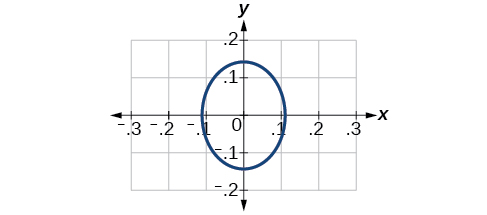36) $$\dfrac{(x-2)^2}{64}+\dfrac{(y-4)^2}{16}=1$$

37) $$\dfrac{(x+3)^2}{9}+\dfrac{(y-3)^2}{9}=1$$

Center $$(-3,3)$$, Vertices $$(0,3)$$, $$(-6,3)$$, $$(-3,0)$$, $$(-3,6)$$. Focus $$(-3,3)$$

Note that this ellipse is a circle. The circle has only one focus, which coincides with the center.38) $$\dfrac{x^2}{2}+\dfrac{(y+1)^2}{5}=1$$

39) $$4x^2-8x+16y^2-32y-44=0$$

Center $$(1,1)$$, Vertices $$(5,1)$$, $$(-3,1)$$, $$(1,3)$$, $$(1,-1)$$. Foci $$(1,1+4\sqrt{3})$$, $$(1,1-4\sqrt{3})$$40) $$x^2-8x+25y^2-100y+91=0$$

41) $$x^2+8x+4y^2-40y+112=0$$

Center $$(-4,5)$$, Vertices $$(-2,5)$$, $$(-6,4)$$, $$(-4,6)$$, $$(-4,4)$$. Foci $$(-4+\sqrt{3}, 5)$$, $$(-4-4\sqrt{3}, 5)$$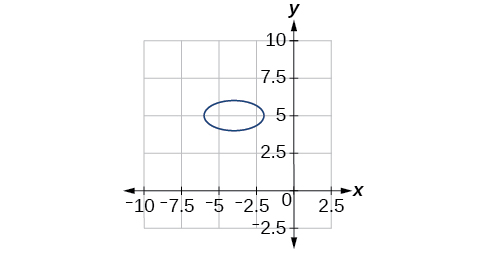42) $$64x^2+128x+9y^2-72y-368=0$$

43) $$16x^2+64x+4y^2-8y+4=0$$

Center $$(-2,1)$$, Vertices $$(0,1)$$, $$(-4,1)$$, $$(-2,5)$$, $$(-2,-3)$$. Foci $$(-2,1+2\sqrt{3})$$, $$(-2,1-2\sqrt{3})$$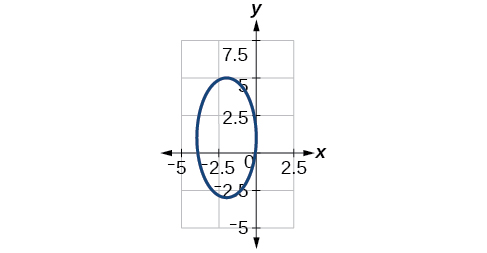44) $$100x^2+1000x+y^2-10y+2425=0$$

45) $$4x^2+16x+4y^2+16y+16=0$$

Center $$(-2,2)$$, Vertices $$(0,-2)$$, $$(-4,-2)$$, $$(-2,0)$$, $$(-2,-4)$$. Focus $$(-2,-2)$$For the exercises 46-51, use the given information about the graph of each ellipse to determine its equation.

46) Center at the origin, symmetric with respect to the $$x$$- and $$y$$-axes, focus at $$(4,0)$$ and point on graph $$(0,3)$$.

47) Center at the origin, symmetric with respect to the $$x$$- and $$y$$-axes, focus at $$(0,-2)$$ and point on graph $$(5,0)$$.

$$\dfrac{x^2}{25}+\dfrac{y^2}{29}=1$$

48) Center at the origin, symmetric with respect to the $$x$$- and $$y$$-axes, focus at $$(3,0)$$, and major axis is twice as long as minor axis.

49) Center $$(4,2)$$; vertex $$(9,2)$$; one focus: $$(4+2\sqrt{6}, 2)$$

$$\dfrac{(x-4)^2}{25}+\dfrac{(y-2)^2}{1}=1$$

50) Center $$(3,5)$$; vertex $$(3,11)$$; one focus: $$(3, 5+4\sqrt{2})$$

51) Center $$(-3,4)$$; vertex $$(1,4)$$; one focus: $$(-3+2\sqrt{3}, 4)$$

$$\dfrac{(x+3)^2}{16}+\dfrac{(y-4)^2}{4}=1$$

For the exercises 52-56, given the graph of the ellipse, determine its equation.

52)53)$$\dfrac{x^2}{81}+\dfrac{y^2}{9}=1$$

54)55)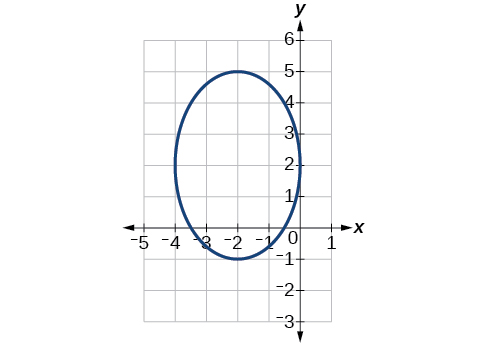$$\dfrac{(x+2)^2}{4}+\dfrac{(y-2)^2}{9}=1$$

56)### Extensions

For the exercises 57-61, find the area of the ellipse. The area of an ellipse is given by the formula $$\text {Area}=a\cdot b\cdot \pi$$

57) $$\dfrac{(x-3)^2}{9}+\dfrac{(y-3)^2}{16}=1$$

$$\text {Area} = 12\pi$$ square units

58) $$\dfrac{(x+6)^2}{16}+\dfrac{(y-6)^2}{36}=1$$

59) $$\dfrac{(x+1)^2}{4}+\dfrac{(y-2)^2}{5}=1$$

$$\text {Area} = 2\sqrt{5} \pi$$ square units

60) $$4x^2-8x+9y^2-72y+112=0$$

61) $$9x^2-54x+9y^2-54y+81=0$$

$$\text {Area} = 9\pi$$ square units

### Real-World Applications

62) Find the equation of the ellipse that will just fit inside a box that is $$8$$ units wide and $$4$$ units high.

63) Find the equation of the ellipse that will just fit inside a box that is four times as wide as it is high. Express in terms of $$h$$, the height.

$$\dfrac{x^2}{4h^2}+\dfrac{y^2}{\tfrac{1}{4}h^2}=1$$

64) An arch has the shape of a semi-ellipse (the top half of an ellipse). The arch has a height of $$8$$ feet and a span of $$20$$ feet. Find an equation for the ellipse, and use that to find the height to the nearest $$0.01$$ foot of the arch at a distance of $$4$$ feet from the center.

65) An arch has the shape of a semi-ellipse. The arch has a height of $$12$$ feet and a span of $$40$$ feet. Find an equation for the ellipse, and use that to find the distance from the center to a point at which the height is $$6$$ feet. Round to the nearest hundredth.

$$\dfrac{x^2}{400}+\dfrac{y^2}{144}=1$$. Distance = $$17.32$$ feet

66) A bridge is to be built in the shape of a semi-elliptical arch and is to have a span of $$120$$ feet. The height of the arch at a distance of $$40$$ feet from the center is to be $$8$$ feet. Find the height of the arch at its center.

67) A person in a whispering gallery standing at one focus of the ellipse can whisper and be heard by a person standing at the other focus because all the sound waves that reach the ceiling are reflected to the other person. If a whispering gallery has a length of $$120$$ feet, and the foci are located $$30$$ feet from the center, find the height of the ceiling at the center.

Approximately $$51.96$$ feet

68) A person is standing $$8$$ feet from the nearest wall in a whispering gallery. If that person is at one focus, and the other focus is $$80$$ feet away, what is the length and height at the center of the gallery?

## 10.2: The Hyperbola

In analytic geometry, a hyperbola is a conic section formed by intersecting a right circular cone with a plane at an angle such that both halves of the cone are intersected. This intersection produces two separate unbounded curves that are mirror images of each other.

### Verbal

1) Define a hyperbola in terms of its foci.

A hyperbola is the set of points in a plane the difference of whose distances from two fixed points (foci) is a positive constant.

2) What can we conclude about a hyperbola if its asymptotes intersect at the origin?

3) What must be true of the foci of a hyperbola?

The foci must lie on the transverse axis and be in the interior of the hyperbola.

4) If the transverse axis of a hyperbola is vertical, what do we know about the graph?

5) Where must the center of hyperbola be relative to its foci?

The center must be the midpoint of the line segment joining the foci.

### Algebraic

For the exercises 6-10, determine whether the following equations represent hyperbolas. If so, write in standard form.

6) $$3y^2 + 2x = 6$$

7) $$\dfrac{x^2}{36}-\dfrac{y^2}{9}=1$$

yes $$\dfrac{x^2}{6^2}-\dfrac{y^2}{3^2}=1$$

8) $$5y^2 + 4x^2 = 6x$$

9) $$25x^2 - 16y^2 = 400$$

yes $$\dfrac{x^2}{4^2}-\dfrac{y^2}{5^2}=1$$

10) $$-9x^2+18x+y^2+4y-14=0$$

For the exercises 11-25, write the equation for the hyperbola in standard form if it is not already, and identify the vertices and foci, and write equations of asymptotes.

11) $$\dfrac{x^2}{25}-\dfrac{y^2}{36}=1$$

$$\dfrac{x^2}{5^2}-\dfrac{y^2}{6^2}=1$$; vertices: $$(5,0)$$, $$(-5,0)$$; foci: $$(\sqrt{61},0)$$, $$(-\sqrt{61},0)$$; asymptotes: $$y=\dfrac{6}{5}x$$, $$y=-\dfrac{6}{5}x$$

12) $$\dfrac{x^2}{100}-\dfrac{y^2}{9}=1$$

13) $$\dfrac{y^2}{4}-\dfrac{x^2}{81}=1$$

$$\dfrac{y^2}{2^2}-\dfrac{x^2}{9^2}=1$$; vertices: $$(0,2)$$, $$(0,-2)$$; foci: $$(0,\sqrt{85})$$, $$(0,-\sqrt{85})$$; asymptotes: $$y=\dfrac{2}{9}x$$, $$y=-\dfrac{2}{9}x$$

14) $$9y^2 - 4x^2 = 1$$

15) $$\dfrac{(x-1)^2}{9}-\dfrac{(y-2)^2}{16}=1$$

$$\dfrac{(x-1)^2}{3^2}-\dfrac{(y-2)^2}{4^2}=1$$; vertices: $$(4,2)$$, $$(-2,2)$$; foci: $$(6,2)$$, $$(-4,2)$$; asymptotes: $$y=\dfrac{4}{3}(x-1)+2$$, $$y=-\dfrac{4}{3}(x-1)+2$$

16) $$\dfrac{(y-6)^2}{36}-\dfrac{(x+1)^2}{16}=1$$

17) $$\dfrac{(x-2)^2}{49}-\dfrac{(y+7)^2}{49}=1$$

$$\dfrac{(x-2)^2}{7^2}-\dfrac{(y+7)^2}{7^2}=1$$; vertices: $$(9,-7)$$, $$(-5,-7)$$; foci: $$(2+7\sqrt{2},-7)$$, $$(2-7\sqrt{2},-7)$$; asymptotes: $$y=x-9$$, $$y=-x-5$$

18) $$4x^2-8x-9y^2-72y+112=0$$

19) $$-9x^2-54x+9y^2-54y+81=0$$

$$\dfrac{(x+3)^2}{3^2}-\dfrac{(y-3)^2}{3^2}=1$$; vertices: $$(0,3)$$, $$(-6,3)$$; foci: $$(-3+3\sqrt{2},1)$$, $$(-3-3\sqrt{2},1)$$; asymptotes: $$y=x+6$$, $$y=-x$$

20) $$4x^2-24x-36y^2-360y+864=0$$

21) $$-4x^2+24x+16y^2-128y+156=0$$

$$\dfrac{(y-4)^2}{2^2}-\dfrac{(x-3)^2}{4^2}=1$$; vertices: $$(3,6)$$, $$(3,2)$$; foci: $$(3,4+2\sqrt{5})$$, $$(3,4-2\sqrt{5})$$; asymptotes: $$y=\dfrac{1}{2}(x-3)+4$$, $$y=-\dfrac{1}{2}(x-3)+4$$

22) $$-4x^2+40x+25y^2-100y+100=0$$

23) $$x^2+2x-100y^2-1000y+2401=0$$

$$\dfrac{(y+5)^2}{7^2}-\dfrac{(x+1)^2}{70^2}=1$$; vertices: $$(-1,2)$$, $$(-1,-12)$$; foci: $$(-1,-5+7\sqrt{101})$$, $$(-1,-5-7\sqrt{101})$$; asymptotes: $$y=\dfrac{1}{10}(x+1)-5$$, $$y=-\dfrac{1}{10}(x+1)-5$$

24) $$-9x^2+72x+16y^2+16y+4=0$$

25) $$4x^2+24x-25y^2+200y-464=0$$

$$\dfrac{(x+3)^2}{5^2}-\dfrac{(y-4)^2}{2^2}=1$$; vertices: $$(2,4)$$, $$(-8,4)$$; foci: $$(-3+\sqrt{29},4)$$, $$(-3-\sqrt{29},4)$$; asymptotes: $$y=\dfrac{2}{5}(x+3)+4$$, $$y=-\dfrac{2}{5}(x+3)+4$$

For the exercises 26-30, find the equations of the asymptotes for each hyperbola.

26) $$\dfrac{y^2}{3^2}-\dfrac{x^2}{3^2}=1$$

27) $$\dfrac{(x-3)^2}{5^2}-\dfrac{(y+4)^2}{2^2}=1$$

$$y=\dfrac{2}{5}(x-3)-4$$, $$y=-\dfrac{2}{5}(x-3)-4$$

28) $$\dfrac{(y-3)^2}{3^2}-\dfrac{(x+5)^2}{6^2}=1$$

29) $$9x^2-18x-16y^2+32y-151=0$$

$$y=\dfrac{3}{4}(x-1)+1$$, $$y=-\dfrac{3}{4}(x-1)+1$$

30) $$16y^2+96y-4x^2+16x+112=0$$

### Graphical

For the exercises 31-44, sketch a graph of the hyperbola, labeling vertices and foci.

31) $$\dfrac{x^2}{49}-\dfrac{y^2}{16}=1$$32) $$\dfrac{x^2}{64}-\dfrac{y^2}{4}=1$$

33) $$\dfrac{y^2}{9}-\dfrac{x^2}{25}=1$$34) $$81x^2-9y^2=1$$

35) $$\dfrac{(y+5)^2}{9}-\dfrac{(x-4)^2}{25}=1$$36) $$\dfrac{(x-2)^2}{8}-\dfrac{(y+3)^2}{27}=1$$

37) $$\dfrac{(y-3)^2}{9}-\dfrac{(x-3)^2}{9}=1$$38) $$-4x^2-8x+16y^2-32y-52=0$$

39) $$x^2-8x-25y^2-100y-109=0$$40) $$-x^2+8x+4y^2-40y+88=0$$

41) $$64x^2+128x-9y^2-72y-656=0$$42) $$16x^2+64x-4y^2-8y-4=0$$

43) $$-100x^2+1000x+y^2-10y-2575=0$$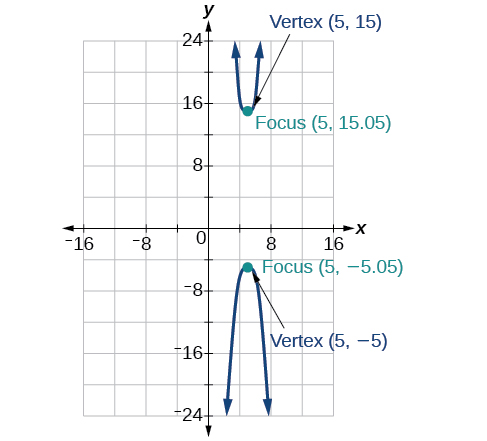44) $$4x^2+16x-4y^2+16y+16=0$$

For the exercises 45-50, given information about the graph of the hyperbola, find its equation.

45) Vertices at $$(3,0)$$ and $$(-3,0)$$ and one focus at $$(5,0)$$.

$$\dfrac{x^2}{9}-\dfrac{y^2}{16}=1$$

46) Vertices at $$(0,6)$$ and $$(0,-6)$$ and one focus at $$(0,-8)$$.

47) Vertices at $$(1,1)$$ and $$(11,1)$$ and one focus at $$(12,1)$$.

$$\dfrac{(x-6)^2}{25}-\dfrac{(y-1)^2}{11}=1$$

48) Center: $$(0,0)$$; vertex: $$(0,-13)$$$;$one focus: $$(0,\sqrt{313})$$.

49) Center: $$(4,2)$$; vertex: $$(9,2)$$$;$one focus: $$(4+\sqrt{26},2)$$.

$$\dfrac{(x-4)^2}{25}-\dfrac{(y-2)^2}{1}=1$$

50) Center: $$(3,5)$$; vertex: $$(3,11)$$ ; one focus: $$(3,5+2\sqrt{10})$$.

For the exercises 51-,55 given the graph of the hyperbola, find its equation.

51)$$\dfrac{y^2}{16}-\dfrac{x^2}{25}=1$$

52)53)$$\dfrac{y^2}{9}-\dfrac{(x+1)^2}{9}=1$$

54)55)$$\dfrac{(x+3)^2}{25}-\dfrac{(y+3)^2}{25}=1$$

### Extensions

For the exercises 56-60, express the equation for the hyperbola as two functions, with $$y$$ as a function of $$x$$. Express as simply as possible. Use a graphing calculator to sketch the graph of the two functions on the same axes.

56) $$\dfrac{x^2}{4}-\dfrac{y^2}{9}=1$$

57) $$\dfrac{y^2}{9}-\dfrac{x^2}{1}=1$$

$$y(x)=3\sqrt{x^2 +1}$$, $$y(x)=-3\sqrt{x^2 +1}$$58) $$\dfrac{(x-2)^2}{16}-\dfrac{(y+3)^2}{25}=1$$

59) $$-4x^2-16x+y^2-2y-19=0$$

$$y(x)=1+2\sqrt{x^2 +4x+5}$$, $$y(x)=1-2\sqrt{x^2 +4x+5}$$60) $$4x^2-24x-y^2-4y+16=0$$

### Real-World Applications

For the exercises 61-65, a hedge is to be constructed in the shape of a hyperbola near a fountain at the center of the yard. Find the equation of the hyperbola and sketch the graph.

61) The hedge will follow the asymptotes $$y=x$$ and $$y=-x$$, and its closest distance to the center fountain is $$5$$ yards.

$$\dfrac{x^2}{25}-\dfrac{y^2}{25}=1$$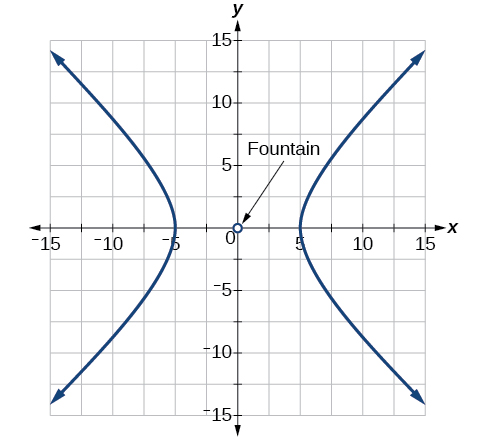62) The hedge will follow the asymptotes $$y=2x$$ and $$y=-2x$$, and its closest distance to the center fountain is $$6$$ yards.

63) The hedge will follow the asymptotes $$y=\dfrac{1}{2}x$$ and $$y=-\dfrac{1}{2}x$$, and its closest distance to the center fountain is $$10$$ yards.

$$\dfrac{x^2}{100}-\dfrac{y^2}{25}=1$$64) The hedge will follow the asymptotes $$y=\dfrac{2}{3}x$$ and $$y=-\dfrac{2}{3}x$$, and its closest distance to the center fountain is $$12$$ yards.

65) The hedge will follow the asymptotes $$y=\dfrac{3}{4}x$$ and $$y=-\dfrac{3}{4}x$$, and its closest distance to the center fountain is $$20$$ yards.

$$\dfrac{x^2}{400}-\dfrac{y^2}{225}=1$$For the exercises 66-70, assume an object enters our solar system and we want to graph its path on a coordinate system with the sun at the origin and the $$x$$-axis as the axis of symmetry for the object's path. Give the equation of the flight path of each object using the given information.
66) The object enters along a path approximated by the line $$y=x-2$$ and passes within $$1$$ au (astronomical unit) of the sun at its closest approach, so that the sun is one focus of the hyperbola. It then departs the solar system along a path approximated by the line